Next: Infinite Body, 1-D, Steady-Periodic Up: Library of Green's Functions Previous: Plate, steady 1-D Helmholtz.

K. D. Cole

In this section, steady-periodic heat conduction is treated. Also called time-harmonic or thermal-wave behavior, this special case is important whenever the causal effect is harmonic in time and has continued long enough for any start-up transients to die out.

Consider a one-dimensional region in which the temperature is sought. The transient temperature distribution satisfies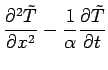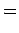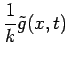(1)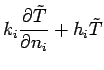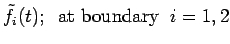Here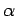is the thermal diffusivity (m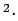s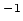),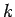is the thermal conductivity (W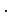m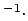K),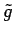is the volume heating (Wm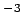), and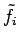is a specified boundary condition. Index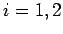represents the boundaries at the limiting values of coordinate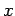. The boundary condition may be one of three types at each boundary: boundary type 1 is specified temperature (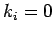and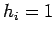); boundary type 2 is specified heat flux (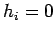); and, boundary type 3 is specified convection where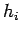is a constant-with-time heat transfer coefficient (or contact conductance).

Since in this section the applications of interest involve steady-periodic heating, the solution is sought in Fourier-transform space, and the solution is interpreted as the steady-periodic response at a single frequency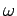. For further discussion of this point see Mandelis (2001, page 2-3). Consider the Fourier transform of the above temperature equations: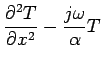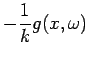(2)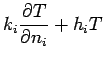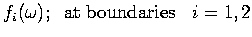Here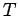is the steady-periodic temperature,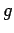is the steady-periodic volume heating,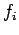is the steady-periodic specified boundary condition, and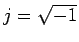.

The temperature will be found with the Fourier-space Green's function, defined by the following equations: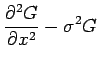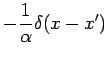(3)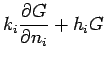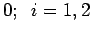(4)

Here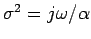and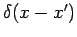is the Dirac delta function. The coefficient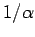preceding the delta function in Eq. (3) provides the 1-D frequency-domain Green's function with units of sm. This is consistent with earlier work with time-domain Green's functions.

If the steady-periodic Green's function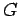is known (given below), then the steady-periodic temperature is given by the following integral equation: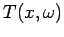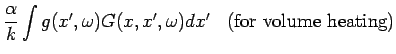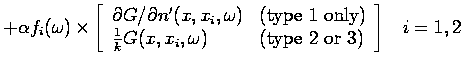(5)

For a derivation of this equation see Beck et al. (1992, pp. 40-43). Next the steady-periodic Green's functions are given for 1-D bodies for cases XIJ.Next: Infinite Body, 1-D, Steady-Periodic Up: Library of Green's Functions Previous: Plate, steady 1-D Helmholtz.

2004-08-10